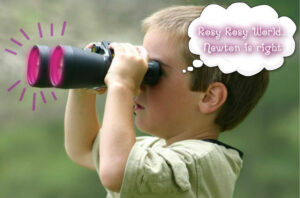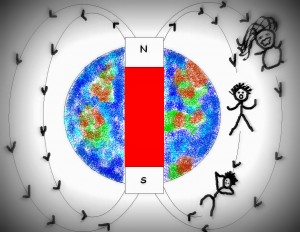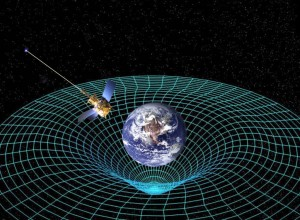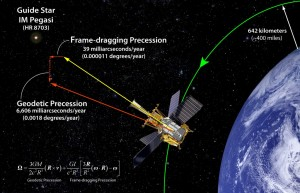| Contact us at durofy@live.com

## The Lessons:

According to our teachers at school, gravitational force is the weak force of attraction between bodies of mass (proportional to m*M and inversely proportional to d2) and gravity exists due to the gravitational force of the earth. But why were we taught about gravity as a FACT and not as a THEORY (published as Newton’s Universal Theory of Gravity)? Surely if it’s a theory there must be limitations, right?

### The Limitations of Newton’s theory:

To begin with, Newton did not hypothesize the cause of gravitational force stating that it’s “enough to know that such a force exists”. Scientists later attributed the cause of this force to ‘gravitons’, which are yet to be found and validated.

Now consider a simple yet famous experiment conducted by Galileo years before Newton presented his theory. He is supposed to have dropped a boulder and a pebble at the same time from the Leaning Tower of Pisa. Assuming that Newton’s theory holds true, as the boulder is heavier, the tug of gravity is more on the boulder. So the boulder should reach theground earlier than the pebble (well, ‘theoretically’). But it was observed that both reached the ground at the same time. But here again being a genius, Newton invented a whole new branch of mathematics (which is surprising flawed as it is based on the laws of infinitesimals and thus not entirely accurate) called CALCULUS to mathematically prove his theory. So accordingly one can argue that when F= (G*mM /d2) and F= ma   => means that though the tug is larger on the boulder, it is heavier, and thus reach the ground exactly at the same time as the pebble. But here is an uncomfortable fact: We are using mathematics developed by NEWTON to prove the theory given by NEWTON.

Also, if the sun’s gravitational force makes all the eight huge planets orbit around itself, it can deviate the orbitting moons, right? Then why doesn’t it happen?

I am not against Newton’s Universal Theory of Gravity (though some not so kind people go to the extent of saying that the title itself- “UNIVERSAL” -has a socialist ring to it), but teaching it as a FACT in schools is definitely not decent.What’s weirder still is saying that the earth is a huge magnet and HENCE gravity exists! Okay, so where are its poles? The North and South poles?! Then according to the Magnetic-Theory, the magnetic lines extend from North Pole to South Pole outside the earth, very much like longitudes that are pushed out into the space. Then like any other body experiencing magnetic force, all matter must be aligning parallel to those imaginary magnetic lines…which is not the case thankfully. :D

So, Einstein comes to the rescue.

## Einstein’s theory of Gravity:This is the only alternative theory of Gravity that is valid in higher number of cases than that of Newton’s universal theory of gravity. It states boldly that there exists a fourth dimension that is related to time (i.e time*sqrt(-1)) in addition to the three dimensions of space. Hence when a massive body is spinning, it makes the four dimensions to wrap and press around it like how a heavy bowling ball in the middle of trampoline would make the trampoline push against it as shown in the picture (Courtesy: NASA). This explains why we are stopped from zooming off into the sky and why the moon still remains faithful to the earth. This is called the geodetic effect.

In Dr. Francis Everitt’s words “like how when an object in honey is stirred it drags the honey around it”, in the same way the massive astronomical objects drag the fabric of space and time around them while spinning. This effect is called frame dragging. Now we know that in the Pisa Tower experiment, the spacetime warping effect pushes both the boulder and the pebble towards the ground with equally. This bizarre theory, apart from covering up the loopholes of Newton’s theory, has also explained ‘universal’(no pun intended) anomalies called gravitational time dilation and gravitational lensing.

## Explanation of Time Dilation and Gravitational lensing:

Gravitational time dilation is something that no normal IQ person has ever completely understood. Let’s give it a try though. It is said that when I move through space, I move through time as well. As the push of spacetime is more near the surface of the earth, time runs slower…and away from the earth it runs faster. So, if I have a twin, having no better work than zooming around the earth in a satellite far away from the surface of the earth, she would return home younger than me. Yikes! This mind-situation is nastily acclaimed as ‘The Twins Paradox’. But hey, time dilation does occur in reality and even the extremely accurate atomic-clocks in our GPS satellites are said to be adjusted and aligned with the earth-time regularly.

Now coming to gravitational lensing, this is a much more widely observed phenomenon. Starlight has been observed to bend around massive bodies rather than going in a straight line passing closely to the surface (we are talking about huge distances i.e. light years here). So our philosophical Newton says that when such a huge amount of gravitational effect (massive bodies remember?) is considered, even photons in the starlight experience gravity and thus bend. But when calculations are made according to this hypothesis, scientists found that the bending results are just half the amount of bending observed. However, calculations according to Einstein’s theory come closer to the observed readings of starlight bending. So how did Einstein explain this phenomenon? Dwell further at your own risk.

In accordance to the time dilation phenomenon, time runs slower at the body’s surface than when away from it. So considering the principles of wave mechanics, light waves follow the fastest path which need not be the shortest path. Thus light rays avoid the slower space near the body and take a longer path (hence a curved path) as it is ‘faster’.

## Probing Gravity:

With utterly minuscule observations being necessary to prove Einstein’s theory, Einstein thought it was impossible to practically prove it. But after four decades of working on the required technology, NASA launched ‘Gravity Probe B’ (a satellite based mission) in 2004 to measure the spacetime curvature near earth. And voilà! In 2011, NASA published results saying that Einstein’s theory is true! It declared the effect of frame dragging of 0.000011 degrees per year and geodetic effect of 0.0018 degrees per year due to time-space warp of the earth.

## The amazingly simple experiment:Four ultra-precise Gyroscopes (devices to record orientation/angle) were mounted on the satellite which was orbiting 400 miles above the earth in a polar orbit. The axis of the gyroscope was designed to point initially towards a star called IM Pegasi. If Newton’s theory was right and no spacetime warping existed, the axis would keep pointing to the star forever as the satellite kept orbiting. But at the end of a year there was a slight change in the orientation of the axis by 6,600 milliarc seconds (0.001833 degrees) clearly due to the geodetic and frame dragging effects. This is illustrated in the figure (Courtesy: NASA).

Thus once again science exceeds itself.

P.S: Try living on a staircase at a point one foot above the ground for a duration of 79 years and you will live longer by 90 billionths of a second! ( According to Einstein. :D )

Share.

### About Author### 5 Comments

1.U mean to say I wasted my time in solving all those Physics problem on gravity in +2? :-O

•Sadly, the point is we will always be tested on Newton’s theory, for atleast half a century to come. So, no, not a waste of time. :D

•Its not in vain. Newton’s theory of gravity works perfectly for many practical purposes here on earth. It only looses ground (pun intended) when used in astronomical scale.

NASA uses only Newton’s theory of gravity to launch a Space Shuttle!

•Ofcourse not in vain. :) They are two THEORIES of gravity. Each is valid in its own set of cases as mentioned in the article, and you are right. But the point is Einstein’s theory is valid in more cases than Newton’s. Einstein’s theory is valid at the surface of the earth as well as astronomical distances away from it. Which SHOWS that NASA could have used either Einstein’s or Newton’s theory to launch the space shuttle. :)

2.Interesting article. Nicely explained